# IB DP Physics: HL复习笔记10.2.6 Orbital Motion, Speed & Energy

### Orbital Motion, Speed & Energy

• Since most planets and satellites have a near circular orbit, the gravitational force FG between the sun or another planet provides the centripetal force needed to stay in an orbit
• Both the gravitational force and centripetal force are perpendicular to the direction of travel of the planet
• Consider a satellite with mass m orbiting Earth with mass M at a distance r from the centre travelling with linear speed v

FG = Fcirc

• Equating the gravitational force to the centripetal force for a planet or satellite in orbit gives: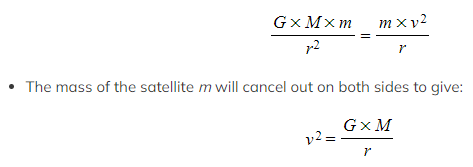• Where:
• v = linear speed of the mass in orbit (m s-1)
• G = Newton's Gravitational Constant
• M = mass of the object being orbited (kg)
• r = orbital radius (m)
• This means that all satellites, whatever their mass, will travel at the same speed v in a particular orbit radius r
• Since the direction of a planet orbiting in circular motion is constantly changing, the centripetal acceleration acts towards the planet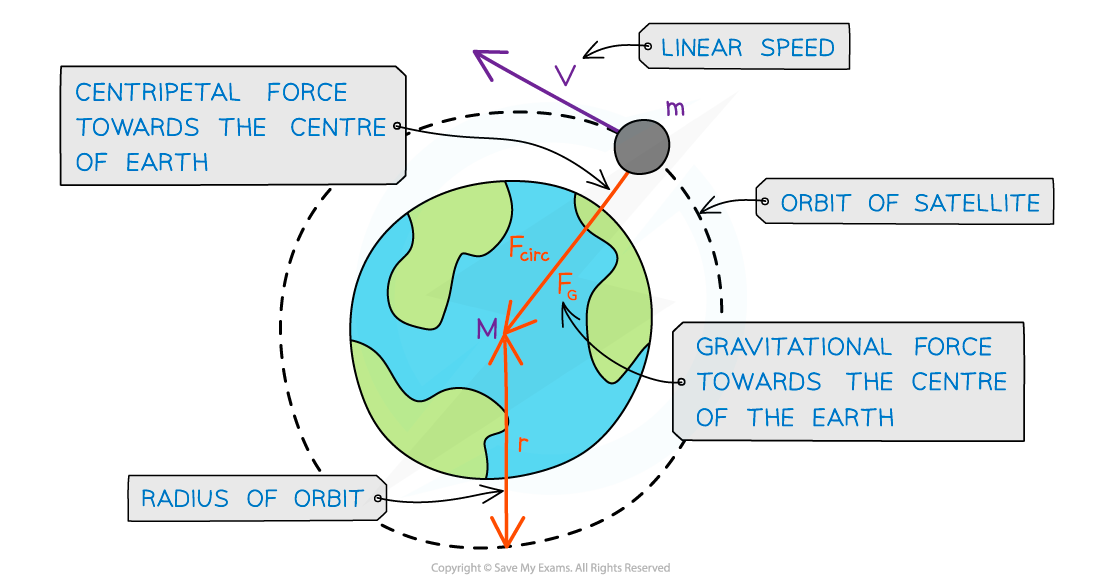A satellite in orbit around the Earth travels in circular motion

#### Time Period & Orbital Radius Relation

• Since a planet or a satellite is travelling in circular motion when in order, its orbital time period T to travel the circumference of the orbit 2πr, the linear speed v is: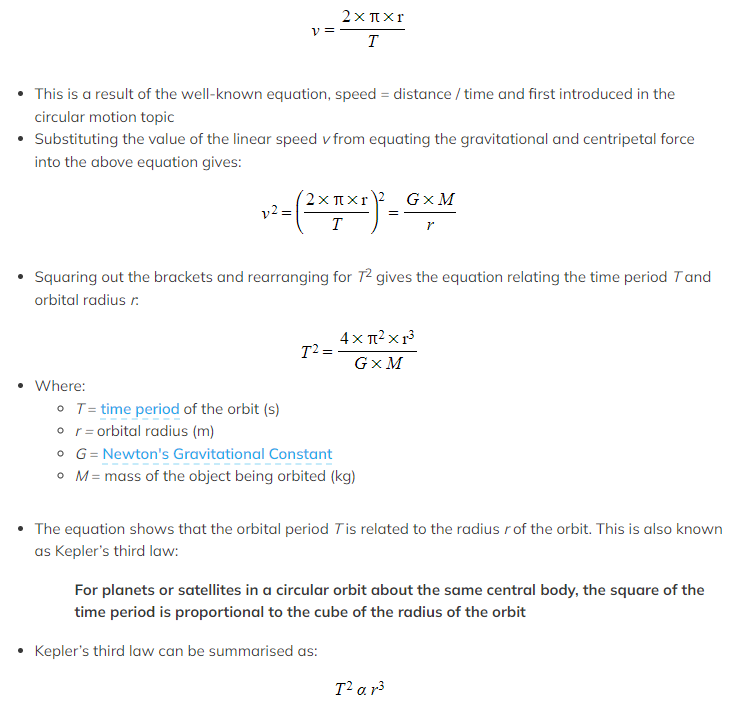Graphical Representation of T2 ∝ r3
• The relationship between T and r can be shown using a logarithmic plot
• T2 ∝ r3
• 2 × log(T) ∝ 3 × log(r)
• The graph of log(T) in years against log(r) in AU (astronomical units) for the planets in our solar system is a straight line graph: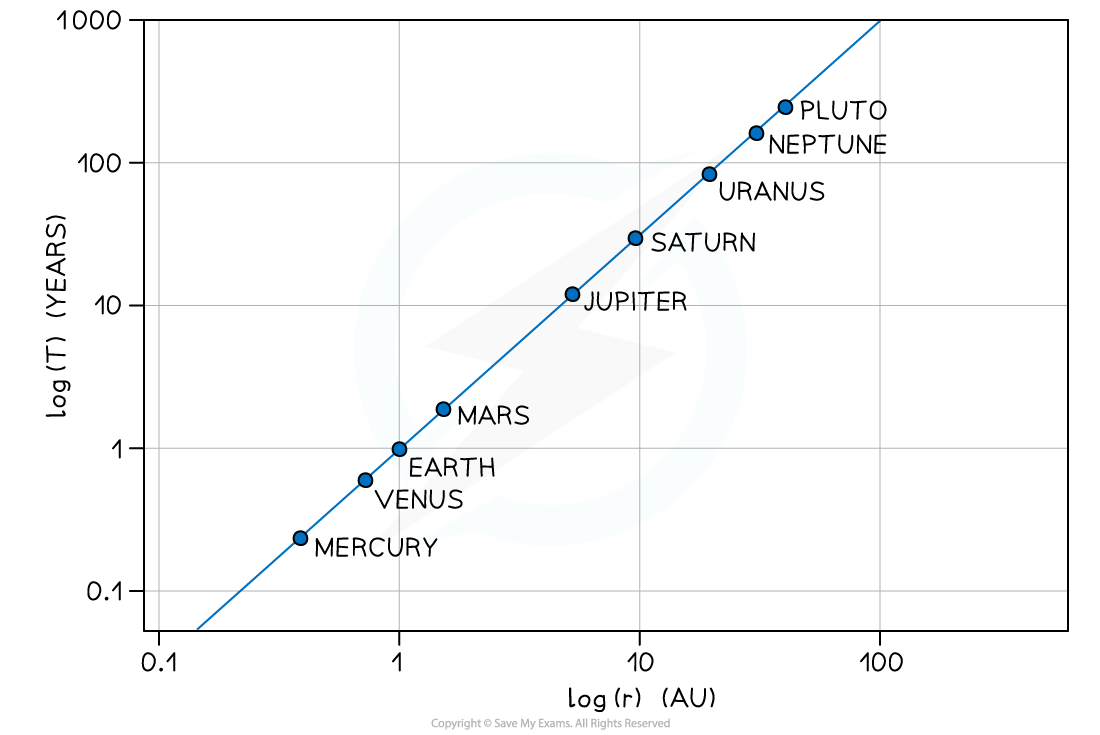• The graph does not go through the origin since it has a negative y-intercept
• Only the log of both T and r will produce a straight line graph

#### Worked Example

A binary star system constant of two stars orbiting about a fixed point B. The star of mass M1 has a circular orbit of radius R1 and mass M2 has a radius of R2. Both have linear speed v and an angular speed ⍵ about B.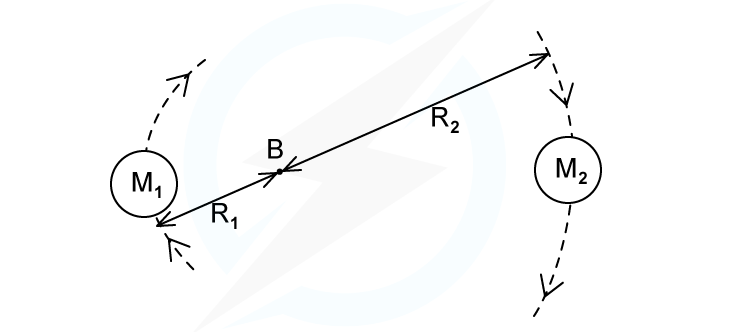State the following formula, in terms of G, M2, R1 and R2

(i) The angular speed ⍵ of M1

(ii) The time period T for each star in terms of angular speed ⍵#### Energy of an Orbiting Satellite

• An orbiting satellite follows a circular path around a planet
• Just like an object moving in circular motion, it has both kinetic energy (KE) and gravitational potential energy (GPE) and its total energy is always constant
• An orbiting satellite's total energy is calculated by: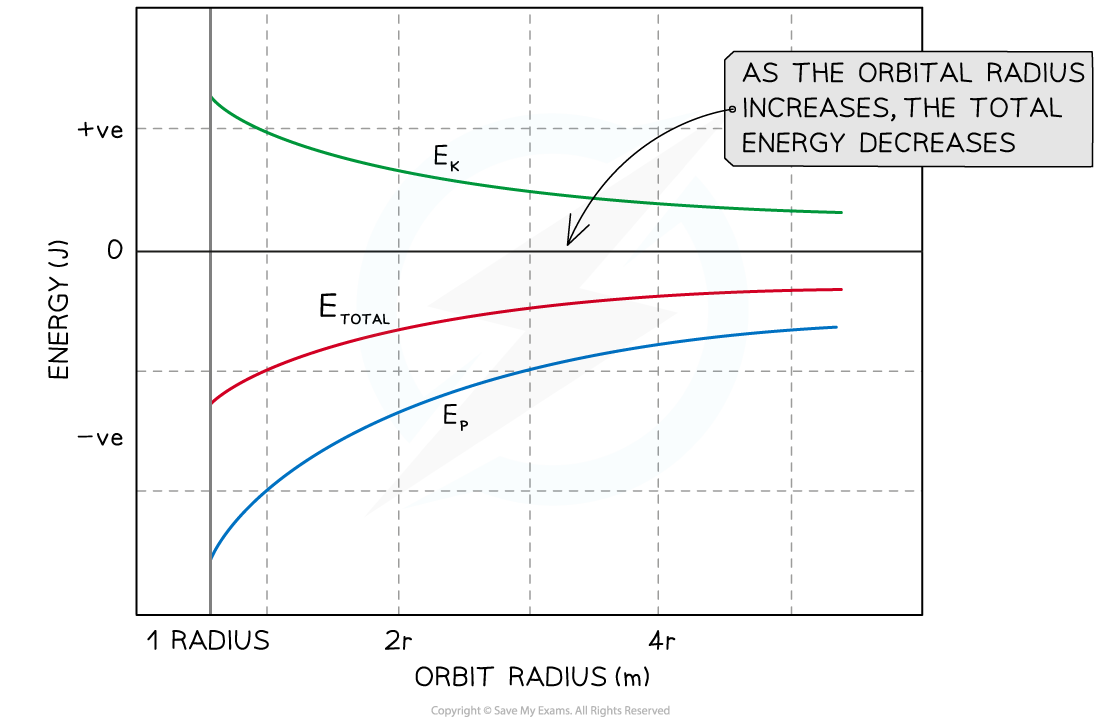A graph showing the kinetic, potential and total energy for a mass at varying orbital distances from a massive body

Total energy = Kinetic energy + Gravitational potential energy

• This means that the satellite's KE and GPE are also both constant in a particular orbit
• If the orbital radius of a satellite decreases its KE increases and its GPE decreases
• If the orbital radius of a satellite increases its KE decreases and its GPE increases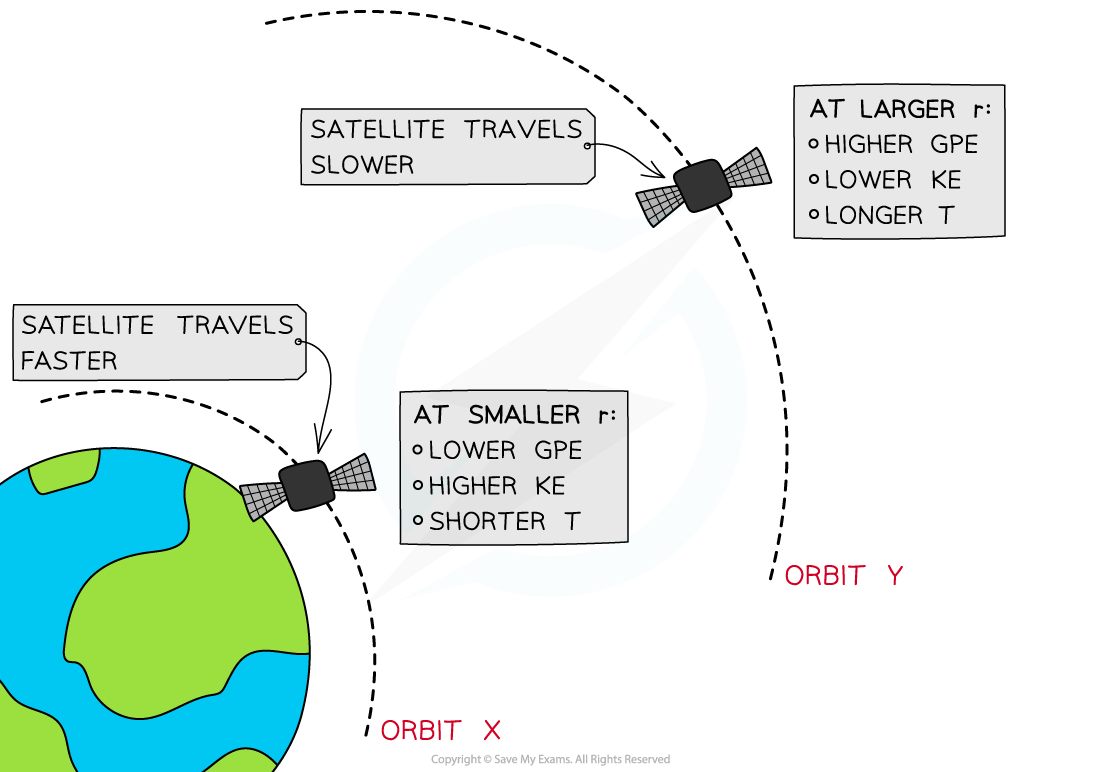At orbit Y, the satellite has greater GPE and less KE than at at orbit X

• A satellite is placed in two orbits, X and Y, around Earth
• At orbit X, where the radius of orbit r is smaller, the satellite has a:
• Larger gravitational force on it
• Higher speed
• Higher KE
• Lower GPE
• Shorter orbital time period, T
• At orbit Y, where the radius of orbit r is larger, the satellite has a:
• Smaller gravitational force on it
• Smaller speed
• Lower KE
• Higher GPE
• Longer orbital time period, T

#### Worked Example

Two satellites A and B, of equal mass, orbit a planet at radii R and 3R respectively. Which one of the following statements is incorrect?

A   A has more kinetic energy and less potential energy than B

B   A has a shorter time period and travels faster than B

C   B has less kinetic energy and more potential energy than A

D   B has a longer time period and travels faster than A

• Since B is at a larger orbital radius (3R instead of R) it has a longer time period since T2 ∝ R3 for an orbiting satellite
• However, satellite B will travel much slower than A
• Its larger orbital radius means the force of gravity will be much lower for B than for A

#### Exam Tip

If you can't remember which way around the kinetic and potential energy increases and decreases, think about the velocity of a satellite at different orbits. When it is orbiting close to a planet, it experiences a larger gravitational pull and therefore orbits faster. Since the kinetic energy is proportional to v2, it, therefore, has higher kinetic energy closer to the planet. To keep the total energy constant, the potential energy must decrease too.

### Orbital Energy Calculations

• A synchronous orbit is:

When an orbiting body has a time period equal to that of the body being orbited and in the same direction of rotation as that body

• These usually refer to satellites (the orbiting body) around planets (the body being orbited)
• The orbit of a synchronous satellite can be above any point on the planet's surface and in any plane
• When the plane of the orbit is directly above the equator, it is known as a geosynchronous orbit

#### Geostationary Orbit

• Many communication satellites around Earth follow a geostationary orbit
• This is sometimes referred to as a geosynchronous orbit
• This is a specific type of orbit in which the satellite:
• Remains directly above the equator
• Is in the plane of the equator
• Always orbits at the same point above the Earth’s surface
• Moves from west to east (same direction as the Earth spins)
• Has an orbital time period equal to Earth’s rotational period of 24 hours
• Geostationary satellites are used for telecommunication transmissions (e.g. radio) and television broadcast
• A base station on Earth sends the TV signal up to the satellite where it is amplified and broadcast back to the ground to the desired locations
• The satellite receiver dishes on the surface must point towards the same point in the sky
• Since the geostationary orbits of the satellites are fixed, the receiver dishes can be fixed too

#### Low Orbits

• Some satellites are in low orbits, which means their altitude is closer to the Earth's surface
• One example of this is a polar orbit, where the satellite orbits around the north and south pole of the Earth
• Low orbits are useful for taking high-quality photographs of the Earth's surface. This could be used for:
• Weather
• Military applications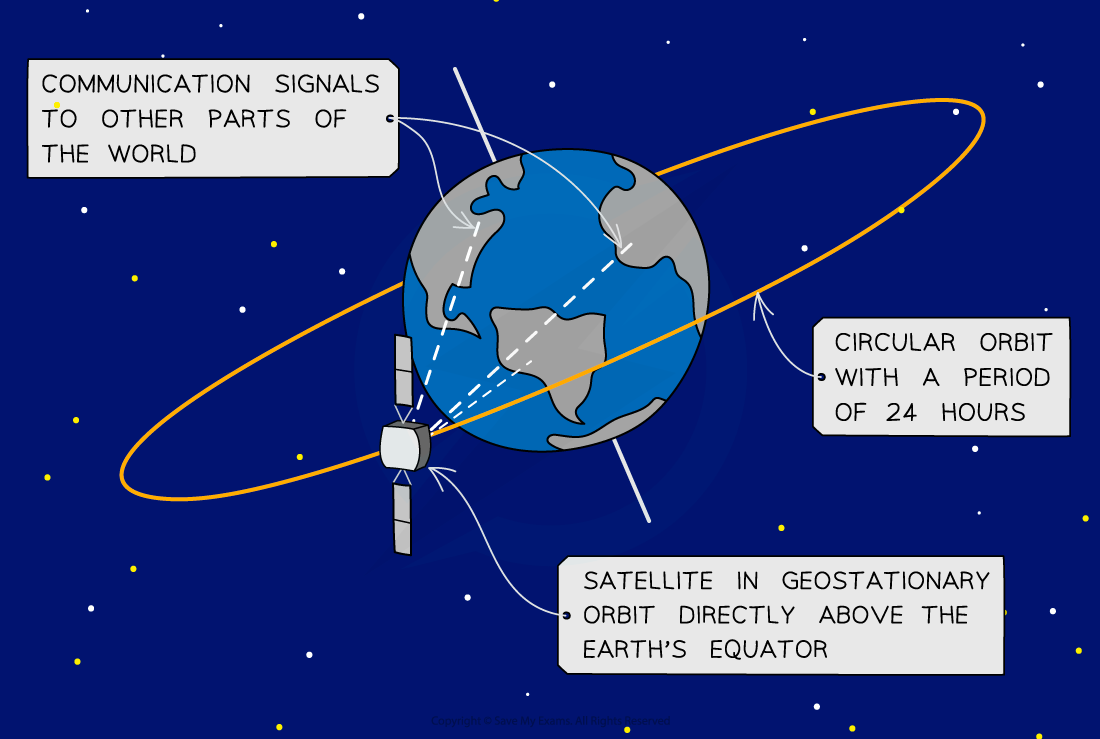Geostationary satellite in orbit

#### Worked Example

Calculate the distance above the Earth's surface that a geostationary satellite will orbit. Mass of the Earth = 6.0 × 1024 kg, Radius of the Earth = 6400 km

#### Orbital Motion

• When a satellite is moving in orbital motion, the velocity of the satellite will be given by:• Where
• ET = The total energy of the satellite (J)
• EP = The potential energy of the satellite (J)
• EK = The kinetic energy of the satellite (J)
• v = the velocity of the satellite (m s-1)
• M = mass of the Earth (or large body being orbited) (kg)
• m = mass of satellite or orbiting object (kg)
• G =  The gravitational constant = 6.67 x 10-11 Nm2kg-2
• r = the orbital radius of the satellite (m)